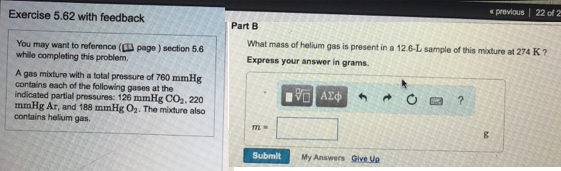Chemistry Practice Problems Ideal Gas Law Molar Mass Practice Problems Solution: A gas mixture with a total pressure of 700 mmHg co...

# Solution: A gas mixture with a total pressure of 700 mmHg contains each of the following gases at the indicated partial pressure: 126 mmHg CO2, 220 mmHg Ar and 188 mmHg O2. The mixture also contain helium gas.Part BWhat mass of helium gas is present in a 12.6 L sample of this mixture at 274 K?Express your answer in grams.

###### Problem

A gas mixture with a total pressure of 700 mmHg contains each of the following gases at the indicated partial pressure: 126 mmHg CO2, 220 mmHg Ar and 188 mmHg O2. The mixture also contain helium gas.

Part B

What mass of helium gas is present in a 12.6 L sample of this mixture at 274 K?View Complete Written Solution

Ideal Gas Law Molar Mass

Ideal Gas Law Molar Mass

#### Q. A wine-dispensing system uses argon canisters to pressurize and preserve wine in the bottle. An argon canister for the system has a volume of 56.8 mL ...

Solved • Fri Oct 05 2018 13:04:00 GMT-0400 (EDT)

Ideal Gas Law Molar Mass

#### Q. A sample of argon gas collected at a pressure of 626 mm Hg and a temperature of 287 K has a mass of 28.1 grams. The volume of the sample is ____ L.

Solved • Thu Aug 09 2018 13:35:38 GMT-0400 (EDT)

Ideal Gas Law Molar Mass

#### Q. A 5.00 L glass bulb with a mass of 1254.49 g is filled with an uknown gas until the pressure is 774 Torr at a temperature of 24.5°C, The mass of bulb ...

Solved • Tue Jul 31 2018 11:48:22 GMT-0400 (EDT)

Ideal Gas Law Molar Mass

#### Q. 3.95 g of an unknown gas at 75°C and 1.05 atm is stored in a 2.55-L flask. What is the density of the gas? What is the molar mass of the gas?

Solved • Wed Jul 25 2018 14:34:00 GMT-0400 (EDT)